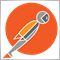Check array to ensure all are same value?13

Hi,

I'm trying to check if all of my strategies in my EA (the ones the user has selected to use) has got a buy or sell signal. Basically, I have an array with the total amount of strategies. For each one, if a buy signal is given, a 1 is placed in the array, sell is 2 and if tno signal is given, its a 0.

My question is, how do I determine if all the values are of the same value, i.e. if all strategies give a buy value, check array to ensure all entries are 1, and there are no 2's or 0's?

It's probably something very simple im overlooking. I am also open to suggestions of easier methods if there are any, rather than using an array.

Thanks!18888

brucey2343: probably something very simple im overlooking. I am also open to suggestions of easier methods if there are any, rather than using an array.
1. Go through each element and see if it is equal to the first. One difference, not all the same.

2. Keep a running count of buys and sells. If you get a count equal to "total amount of strategies," then they all must be the same.279

brucey2343:

Hi,

I'm trying to check if all of my strategies in my EA (the ones the user has selected to use) has got a buy or sell signal. Basically, I have an array with the total amount of strategies. For each one, if a buy signal is given, a 1 is placed in the array, sell is 2 and if tno signal is given, its a 0.

My question is, how do I determine if all the values are of the same value, i.e. if all strategies give a buy value, check array to ensure all entries are 1, and there are no 2's or 0's?

It's probably something very simple im overlooking. I am also open to suggestions of easier methods if there are any, rather than using an array.

Thanks!

Like William says, check all the values in the array. But to speed things up, exit the loop as soon as a different value is encountered?

Something like this (not checked)

```int first=myIndicators;
bool allEqual=true;
for(int x=1;x<ArraySize(myIndicators);x++){
if(myIndicators[x]!=first){
allEqual=false;
break;
}

}```

Actually, you probably don't need an array, if all you are wanting is equality of buy/sell. Just stop the process when you find a strategy value not equal to the first one AS the strategies values are determined.

As an aside, in terms of your buy or sell values, 1,2 and 0 for no signal.

I'd consider making the values buy=0, sell=1 and maybe -1 for no signal for some consistency in your programming ?

Because, order operation constants OP_BUY and OP_SELL are 0 and 1.

A.2380

```template <typename T>
bool all(T &array[])
{
T last_value = NULL;
int size = ArraySize(array);
for (int i=0; i<size; i++) {
if (i == 0) {
last_value = array[i];
continue;
}
if (array[i] != last_value)
return false;
}
return true;
}```